## Basic Econometrics Chapter 7, Problem 21

Question: Consider the following demand function for money in the United States for the period 1980-1998:

where M = real money demand, using the M2 definition of money

Y = real GDP

r = interest rate

To estimate the above demand for money function, you are given the data in Table 7.10.

Note: To convert nominal quantities into real quantities, divide M and GDP by CPI. There is no need to divide the interest rate variable by CPI. Also, note that we have given two interest rates, a short-term rate as measured by the 3-month treasury bill rate and the long-term rate as measured by the yield on the 30-year treasury bond, as prior empirical studies have used both types of interest rates.

TABLE 7.10

Demand for Money in the United States, 1980-1998

Source: Economic Report of the President, 2000, Tables B-1, B-58, B-67, B-71.

Notes: GDP: gross domestic product (\$ billions).

M2: M2 money supply.

CPI: Consumer Price Index (1982­-1984 = 100).

LTRATE: long-term interest rate (30-year Treasury bond).

TBRATE: three-month Treasury bill rate (% per annum).

a. Given the data, estimate the above demand function. What are the income and interest rate elasticities of demand for money?

b. Instead of estimating the above demand function, suppose you were to fit the function

How would you interpret the results? Show the necessary calculations.

c. How will you decide which is a better specification? (Note: A formal statistical test will be given in Chapter 8.)

Solution:

Given information:

The demand function for money can be written as follows: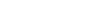…… (1)

Here

Table -1 shows the data of Country U’s demand for money (1980 – 1998) as follows:

(a)

Table -2 shows the estimated result for short-term bond as follows:

From Table -2, the regression equation for short term can be written as follows: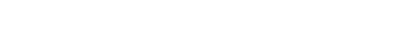…… (2)

Table -3 shows the estimated result for long-term bond as follows:

The regression result for 30-year long–term bond can be written as follows: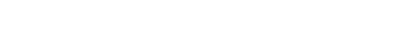…… (3)

From Equations (2) and (3), the value of income elasticity is (0.5243 or 0.4946) and the interest rate elasticities are (-0.0255 or -0.0516). Here, the short-term interest rate (TBrate) gives a better statistical significant in this model.

(b)

The demand function is as follows: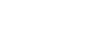…… (4)

Here, the ratio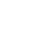represents the cost of holding money. It does not yield more interest income.

Table -4 shows the estimated result for the long term as follows:

The log regression result can be written as follows:…… (5)

Table -5 shows the estimated result for a long-term bond as follows:…… (6)

Both Equations (5) and (6) are bi–variate regression as per expectation K which is statistically significant to the interest rate. The results are more sensitive in the long-term interest rate than the short-term interest rate. Thus, the value of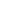in the long-term interest rate gives a better value than the short-term rate.

F-test:

The formula for F-test is represented as follows:…… (7)

Here

RSS R is the residual sum of squares of the restricted regression.

RSS UR is the residual sum of squares of the unrestricted regression.

q is the number of linear restrictions.

n is the number of observations.

k is the number of parameters in the restricted regression.

The value of the F-test can be calculated using Equation (6) as follows:

Hence, the F-statistic is 10.6920.

In this case, it is observed that the result is based on the long-term rate that is similar. Models (2) and (3) are unrestricted and models (4) and (5) are restricted. Here, the dependent variables in the two models are not the same and there is only one restriction variable, which implies that the coefficient of variable Y in Model (1) is unity.

The critical F-value is 4.49 at 5% significance level. Therefore, based on the F-statistic, the model can be rejected, as it defines that the real income elasticity is smaller than unity.